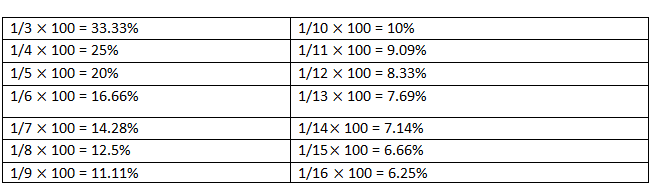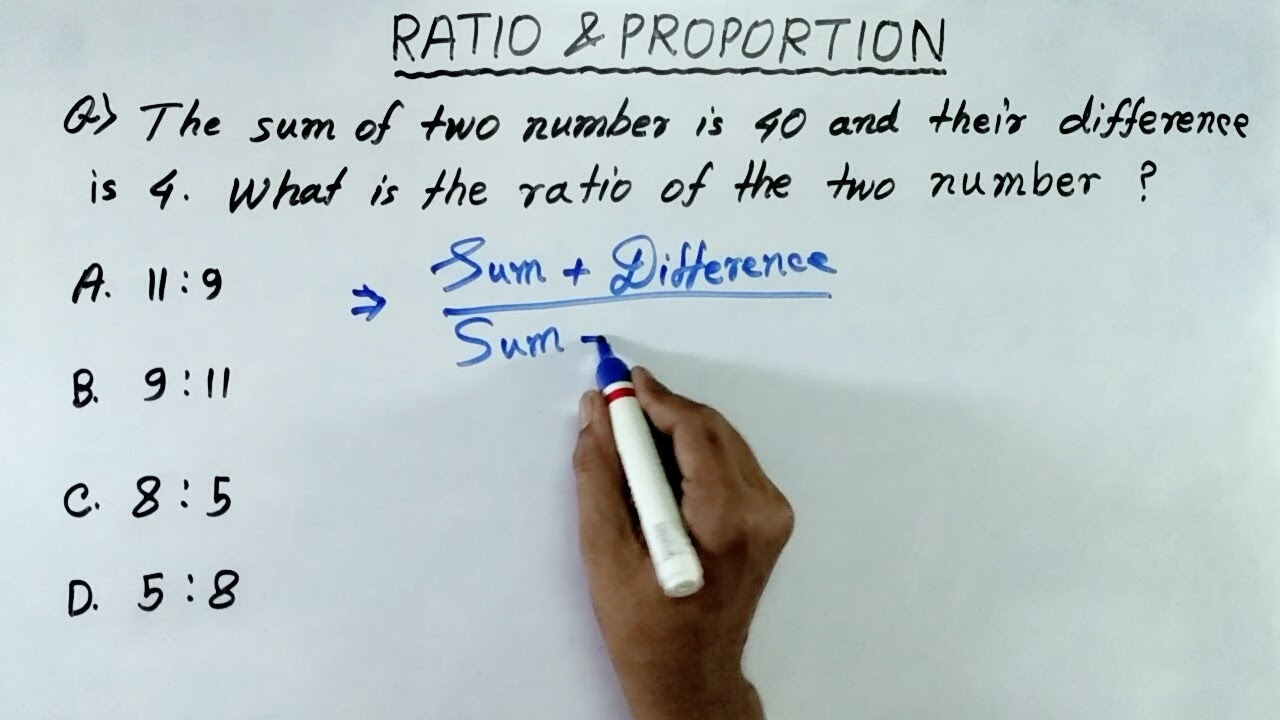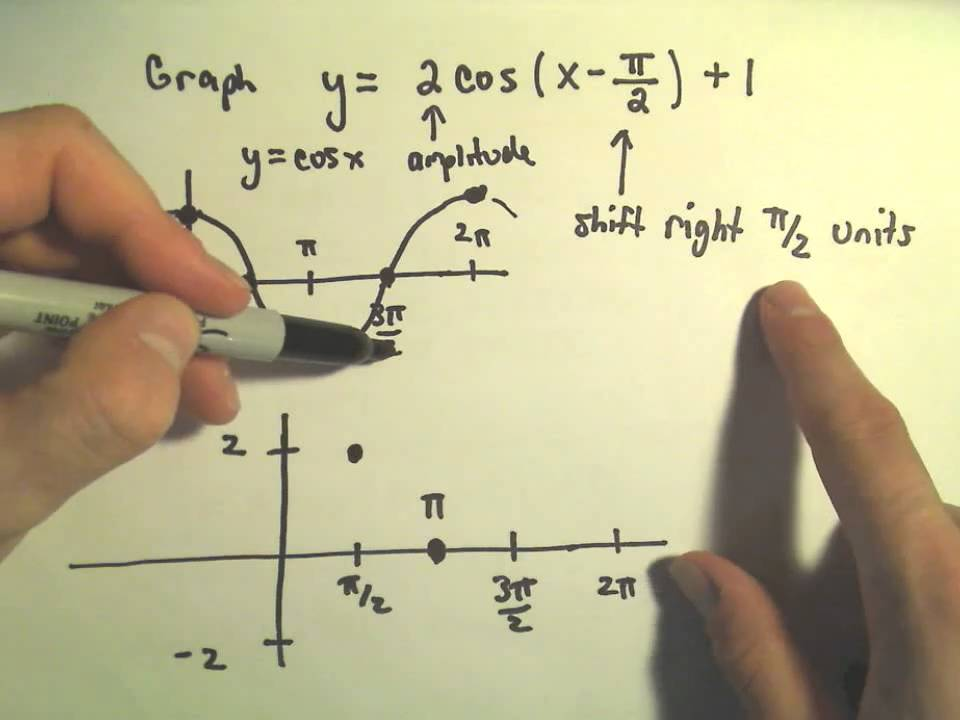SSC-CGL 2020 Quantitative Aptitude (Last minute revison) - Study24x7# Welcome Back

or

By Registering, you agree to our and of use.

# SSC-CGL 2020 Quantitative Aptitude (Last minute revison)

Published on 15 February 2020SSC CGL 2020: Quantitative Aptitude

SSC CGL new vacancy 2020 has been out.

One of the most scoring topics for SSC CGL 2020 is quantitative aptitude.

Mock test in Hindi can also help you to practice more and score well.

## SSC CGL 2020: Percentage

Percentage (%) is a fraction or ratio where denominator is 100.

1. n% of x means

( x * n)/100

Example: 20% of 150 is (20*150)/100 =30

2. n% of x= x% of n

Example: 20% of 5= 5% of 20

(5*20)/100= (20*5)/100=1

3. find what percentage of x is n

(n / x)*100

4. If x is increased/decreased by n, then percentage

x (1+ n/100)

5. Percentage change in value= (change/initial value)*100

6. If price of a commodity increased/decreased by N%, then

change in consumption without changing the expenditure

will be:

(N/ (100 + N)) * 100 %

7. If the Population (P) is increasing with rate R% annually

then,

Population after n years = P*(1 + R/100) n

Population before n years = P/ (1 + R/100 ) n

8. If price (P) of a commodity depreciates R% annually then,

Price of commodity after n years = P*(1 - R/100) n

Price of commodity n years ago = P/ (1 - R/100) nSSC CGL 2020: Ratio and Proportion

Ratio(x/y) is the relation between two or more values of same

kind. Where x is antecedent and y is consequent.

• In ratio order is important. For example: x: y is not same

as y: x.

• If same number is multiplied or divided in each term of

ratio, then ratio will remain unchanged.

• Proportion (::) is when ratio of first and second element is equal

to third and fourth element.

A: B= C: D

For example: 4:10:: 8: 20=2/5

• Fourth Proportional (D) = (B*C)/ A

• If second term is equal to third term A: B:: B:C then,

Third proportional (C) = B 2 /A

• Mean proportional between A and B= √AB
• Duplicate ratio of A and B = A 2 : B 2SSC CGL 2020: Speed, Distance & amp; Time

Measurements:

Speed in km/hr or m/sec or miles/ hr.

Distance in Km or meter or miles.

Time is in hours or minutes or seconds.

Conversions:

Km/hr to meter/ sec = speed in km/hr* 5/18

Meter/sec to km/hr= speed in m/sec* 18/5Formulas:

1. Distance= Speed X Time

2. Speed=Distance/Time

3. Time= Distance/ Speed

4. If object is moving with x and y speed, then

Average speed = (2xy)/(x + y)

5. When two objects are moving in same direction with x and

y speed then

Relative speed = difference of x and y

6. When two objects are moving in different direction with x

and y.

then,

Relative speed= addition of x and y

7. If time taken by objects is same, then

Average speed= (x + y)/2

8. If distance covered by objects is same, then

Average speed= 2x*y/ (x + y)

9. If two people moving between two points A and Bin

opposite direction by crossing each other, then speed of

them will be:

Speed of A/ Speed of B=√Time taken by B/ Time taken by A

10. Calculation of least time taken to meet in circular race

is LCM (T1, T2, T3…… Tn) i.e. Least common Multiple.